Trigonometric values for Any Angle: First Principles

what you'll learn...

Trigonometric Values : First Principles

»  The angle $\omega$$\omega$ is measured from positive x-axis
→  x and y coordinates takes sign.
Quickly follow the sign of x and y projections to find sign of trigonometric values. No need to memorize.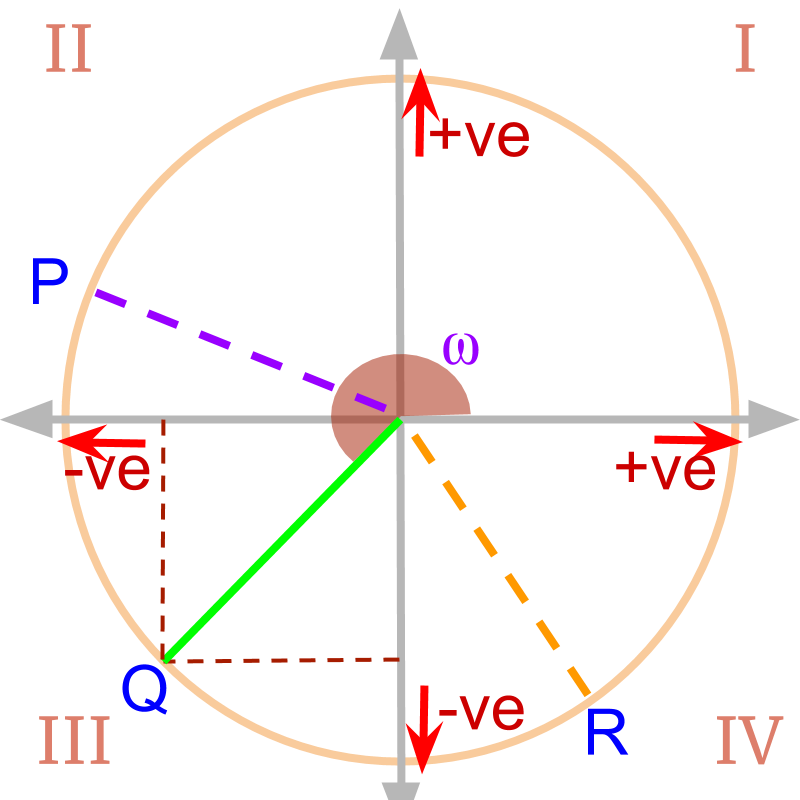»  $P$$P$ is in the second quadrant
→  -ve x projection
→  +ve y projection

»  $Q$$Q$ is in third quadrant
→  -ve: both x and y projections

»  $R$$R$ is in fourth quadrant
→  +ve x projection
→  -ve y projection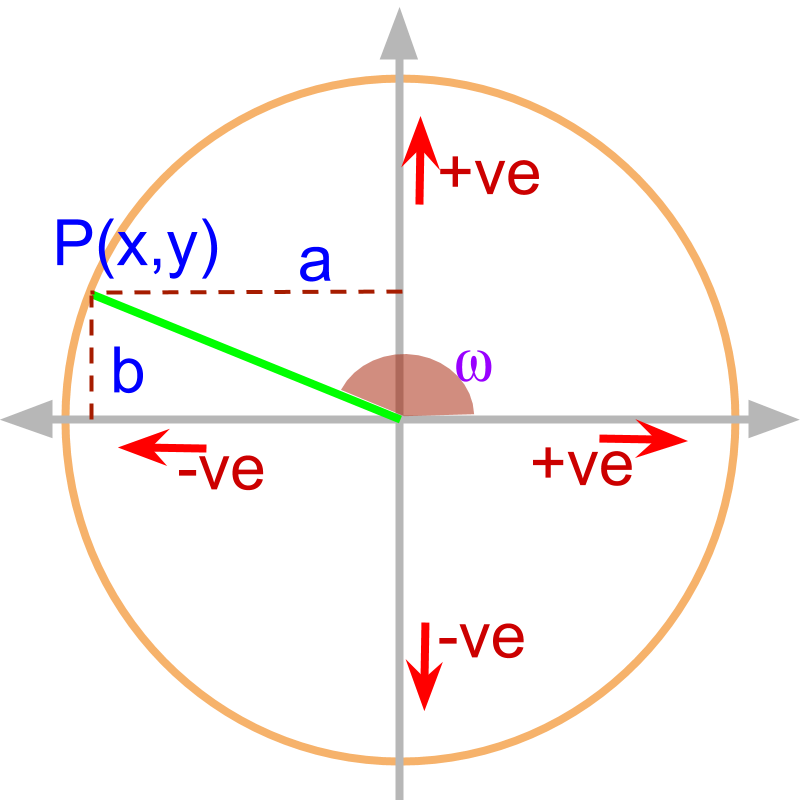The angle $\omega$$\omega$ is shown in the figure. The magnitude of projections on x axis and y axis is given as $a$$a$ and $b$$b$.

It is noted that the projection along $x-$$x -$axis is $-a$$- a$ and so

$\mathrm{tan}\theta =\frac{b}{-a}$$\tan \theta = \frac{b}{- a}$.

The trigonometric ratios are computed from the x and y axis projections.
Note that the projections are given for point on unit circle. so $\sqrt{{a}^{2}+{b}^{2}}=1$$\sqrt{{a}^{2} + {b}^{2}} = 1$.

$\mathrm{sin}\omega =\frac{b}{1}$$\sin \omega = \frac{b}{1}$

$\mathrm{cos}\omega =\frac{-a}{1}$$\cos \omega = \frac{- a}{1}$

$\mathrm{tan}\omega =\frac{b}{-a}$$\tan \omega = \frac{b}{- a}$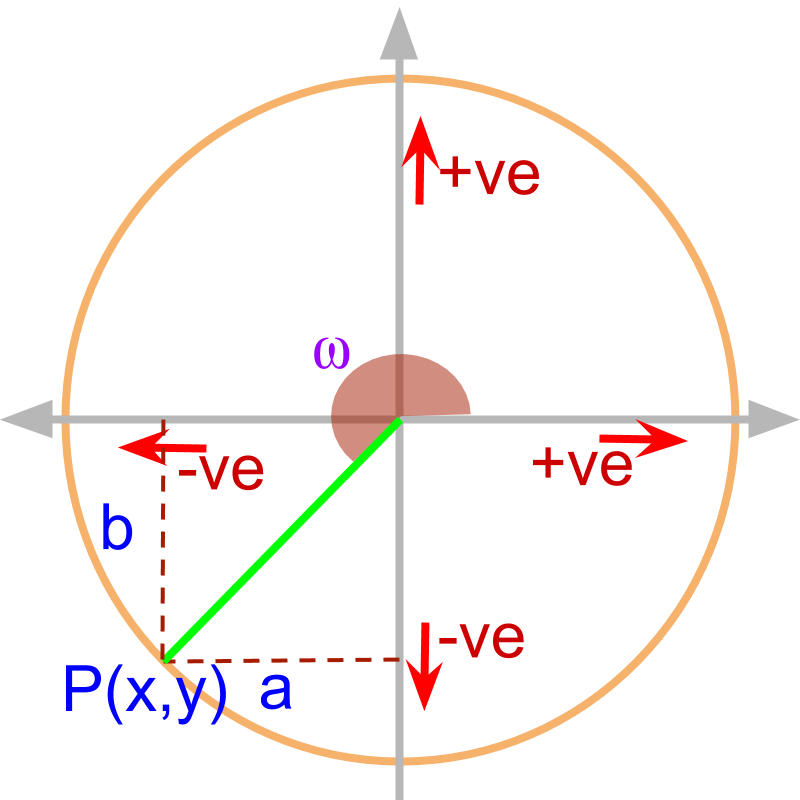The angle $\omega$$\omega$ is shown in the figure. The magnitude of projections on x axis and y axis is given as a and b.

Considering the projections along x axis and y axis as $-a$$- a$ and $-b$$- b$,

$\mathrm{tan}\omega =\frac{-b}{-a}$$\tan \omega = \frac{- b}{- a}$

Note that the sign of the numerator and denominator provide information as to if the angle is in first quadrant or 3rd quadrant.

The trigonometric ratios are computed from the x and y axis projections.
Note that the projections are given for point on unit circle. so $\sqrt{{a}^{2}+{b}^{2}}=1$$\sqrt{{a}^{2} + {b}^{2}} = 1$.

$\mathrm{sin}\omega =\frac{-b}{1}$$\sin \omega = \frac{- b}{1}$

$\mathrm{cos}\omega =\frac{-a}{1}$$\cos \omega = \frac{- a}{1}$

$\mathrm{tan}\omega =\frac{-b}{-a}$$\tan \omega = \frac{- b}{- a}$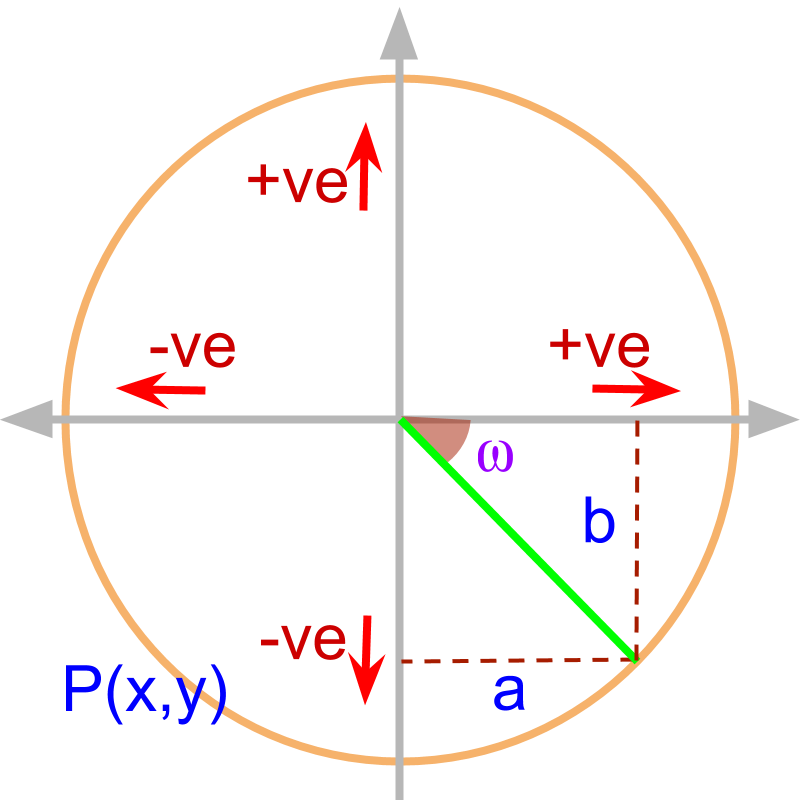The angle $\omega$$\omega$ is negative and is shown in the figure. The magnitude of projections on x axis and y axis is given as a and b.

Considering the projections on x axis and y axis as $a$$a$ and $-b$$- b$, $\mathrm{tan}\omega =\frac{-b}{a}$$\tan \omega = \frac{- b}{a}$

The trigonometric ratios are computed from the x and y axis projections.
Note that the projections are given for point on unit circle. so $\sqrt{{a}^{2}+{b}^{2}}=1$$\sqrt{{a}^{2} + {b}^{2}} = 1$.

$\mathrm{sin}\omega =\frac{-b}{1}$$\sin \omega = \frac{- b}{1}$

$\mathrm{cos}\omega =\frac{a}{1}$$\cos \omega = \frac{a}{1}$

$\mathrm{tan}\omega =\frac{-b}{a}$$\tan \omega = \frac{- b}{a}$

large angle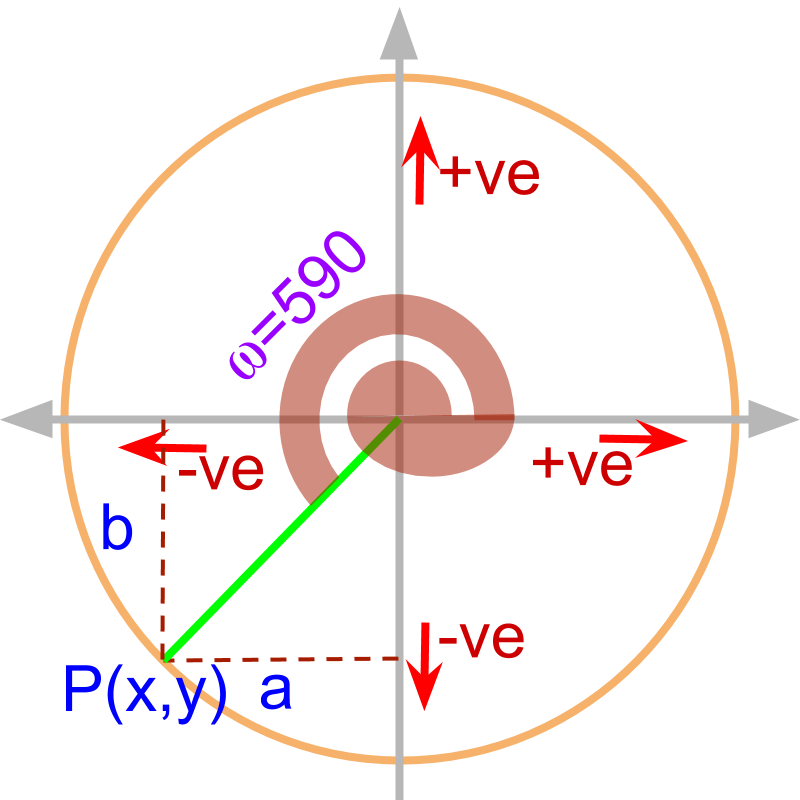The angle omega equals ${590}^{\circ }$${590}^{\circ}$ is shown in the figure. The magnitude of projections on x axis and y axis is given as a and b.

${\mathrm{tan}590}^{\circ }=\frac{-b}{-a}$$\tan {590}^{\circ} = \frac{- b}{- a}$

The trigonometric ratios are computed from the x and y axis projections.

$\mathrm{sin}\omega =\frac{-b}{1}$$\sin \omega = \frac{- b}{1}$

$\mathrm{cos}\omega =\frac{-a}{1}$$\cos \omega = \frac{- a}{1}$

$\mathrm{tan}\omega =\frac{-b}{-a}$$\tan \omega = \frac{- b}{- a}$

For any angle the trigonometric values are computed using the projections of point on unit circle at the given angle on to x and y axis.

summary

First Principles to find Trigonometric Ratios for any Angle: For the given angle, find the projections of point on unit circle at the given angle on to x and y axes. The projections are signed values. The trigonometric ratios are computed as

•  $\mathrm{sin}\omega =y\phantom{\rule{1ex}{0ex}}\text{projection}$$\sin \omega = y \textrm{p r o j e c t i o n}$

•  $\mathrm{cos}\omega =x\phantom{\rule{1ex}{0ex}}\text{projection}$$\cos \omega = x \textrm{p r o j e c t i o n}$

•  $\mathrm{tan}\omega =\frac{y\phantom{\rule{1ex}{0ex}}\text{projection}}{x\phantom{\rule{1ex}{0ex}}\text{projection}}$$\tan \omega = \frac{y \textrm{p r o j e c t i o n}}{x \textrm{p r o j e c t i o n}}$

Outline

It is advised to do the firmfunda version of "basics of Trigonometry" course before doing this.

The outline of material to learn "Advanced Trigonometry" is as follows.
Note: go to detailed outline of Advanced Trigonometry

→   Unit Circle form of Trigonmetric Values

→   Trigonometric Values in all Quadrants

→   Trigonometric Values or any Angles : First Principles

→   Understanding Trigonometric Values in First Quadrant

→   Trigonometric Values in First Quadrant

→   Trigonometric Values of Compound Angles: Geometrical Proof

→   Trigonometric Values of Compound Angles: Algebraic Proof

→   Trigonometric Values of Compound Angles: tan cot

→   Trigonometric Values of Compound Angles: more results